# Survival Analysis with Cox Regression Model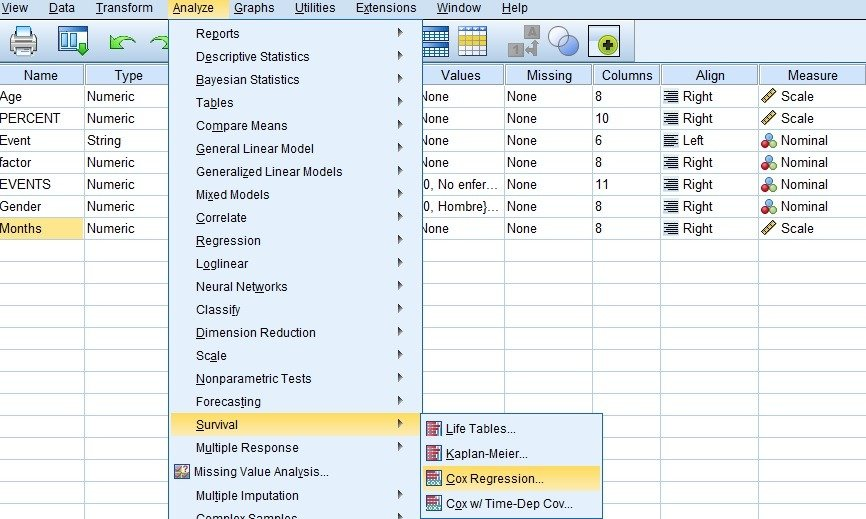# Survival Analysis with Cox Regression Model and Kaplan-Meier Curve

The response dependent variable may be the ‘Follow-up time of patients from the ingestion of a drug until an event occurs in the form of illness or death’, ‘time from discharge to rehospitalization’, ‘time since surgery until having problems again ‘,’ time until having an accident at the company ‘, etc. There is a nuance of censorship as to the times cannot be observed completely, for various reasons: the individual leaves the follow-up, etc. The data will not be distributed normally (asymmetry), so the normality-based model will not be able to take into account.

This time variable is studied with respect to the censored events and with respect to the covariates or explanatory variables of the statistical study, whether continuous, dichotomous or categorical type recoded into dummies (dummy dummy). The latter must be defined as such in the option offered by the SPSS.

## Survival Function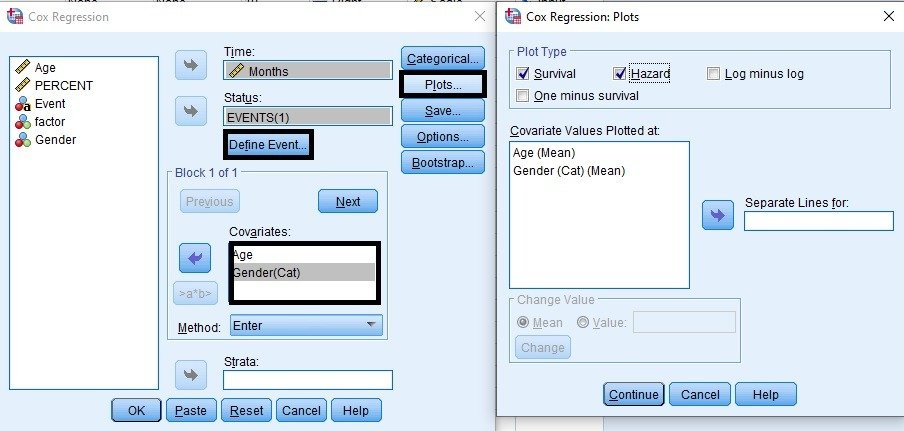### Survival Function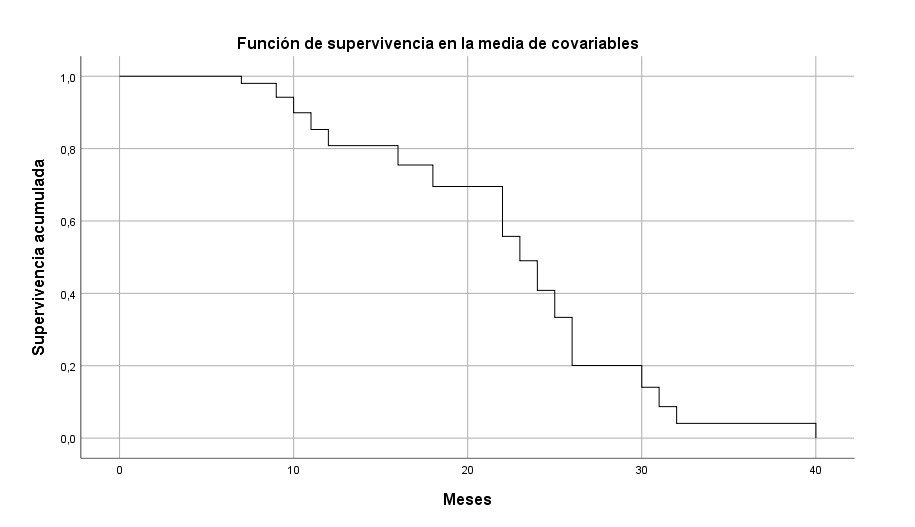What is the probability that an individual is for each time – X months (years) – without an event of interest from our survival analysis? What is the probability that an individual will survive 4 years? In the axis of abscissa, in horizontal, we have the time, in the axis of ordinates, in vertical, we have the probability between 0 and 1.

The Kaplan-Meier estimator calculates the product of 1 minus the risk each individual, understanding as such the ratio between the number of events, and the number of individuals at risk before the event.

### Hazard Function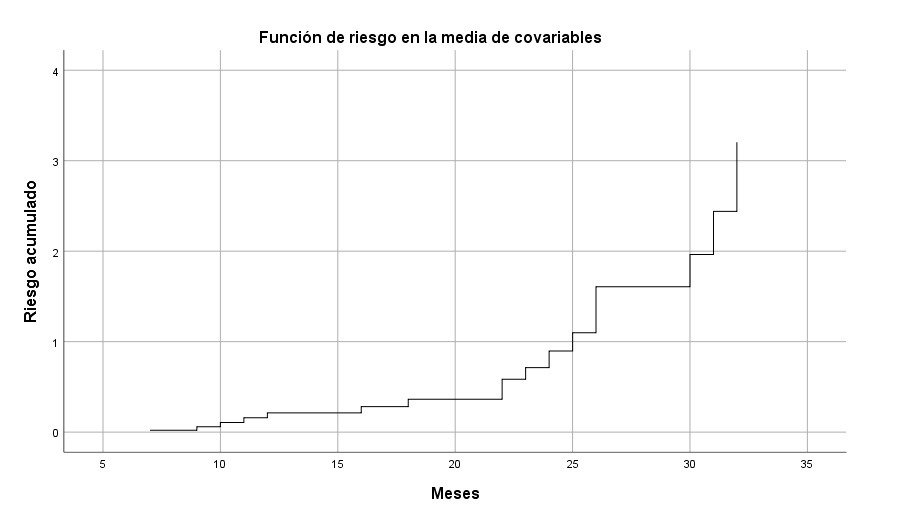## Cox Regression Model

This is the alternative to the standard regression when you have censored events (this is Survival Analysis). It is found that there are significant differences between the treatments in terms of survival time, and this difference can be summarized with a COX regression model, which raises a relationship for the risk between the alternative group, for example men with treatment 2, and men with treatment 1 (reference group), or women with a specific treatment against women in a control group.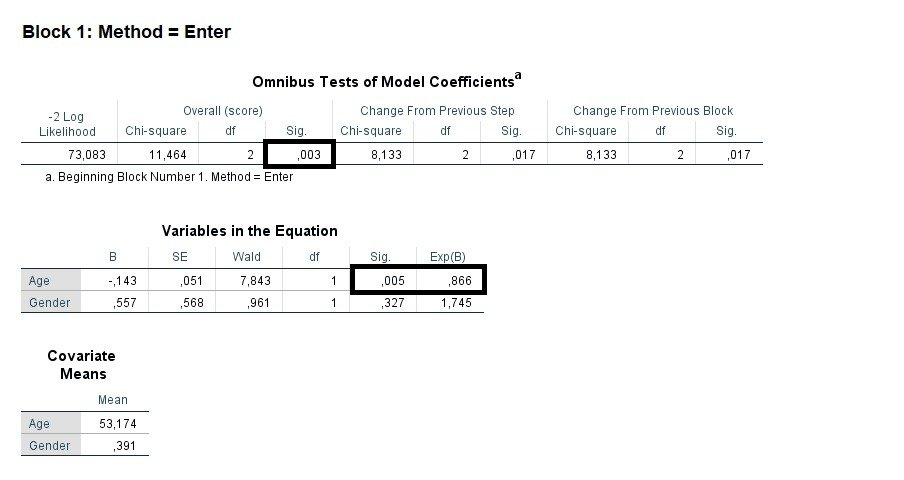In a first step, the model can estimate the values ​​of the coefficients. The Cox regression model is statistically significant (p-value = 0.003 <0.05), as well as the variable Age is significant for the event being studied (Disease), 0.005 <0.05. The value of HR (Hazard Ratio) for this variable is 0.866 <1, that is, at a lower age, higher risk of disease, that is, the HR is interpreted as the increase (> 1) or decrease (<1) of the risk of any of the categories of censored event based on the covariates of the study, while the rest of the explanatory variables remain constant and the risk ratio is always validated as constant.